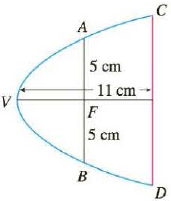Chapter 10.5, Problem 50E

Chapter
Section
Textbook Problem

# A cross-section of a parabolic reflector is shown in the figure. The bulb is located at the focus and the opening at the focus is 10 cm. (a) Find an equation of the parabola. (b) Find the diameter of the opening |CD|, 11 cm from the vertex.a)

To determine

To Find: The equation of the parabola.

Explanation

Given:

The parabola appears as drawn in the below figure.

The opening at the focus is 10cm and distance from the vertex to the CD is 11cm .

Calculation:

Referring to the figure (1), the focus and the vertex are located in the x axis.

The standard equation the parabola is as below.

y2=4px (1)

The focus of the parabola is of the form (p,0) .

Referring to the figure (1) at the point A , the focus of the parabola is (p,5) .

Substitute the value (p,5) for (x,y) in equation (1), to find the value p

b)

To determine

To Find: The diameter of the opening CD.

### Still sussing out bartleby?

Check out a sample textbook solution.

See a sample solution

#### The Solution to Your Study Problems

Bartleby provides explanations to thousands of textbook problems written by our experts, many with advanced degrees!

Get Started

## Additional Math Solutions

#### In Exercises 47-52, find and simplify f(a+h)f(a)h (h 0) for each function. 48. f(x) = 2x2 x + 1

Applied Calculus for the Managerial, Life, and Social Sciences: A Brief Approach

#### Find the x- and y-intercepts of the graph of y=x22x3.

Calculus: An Applied Approach (MindTap Course List)

#### Find the derivative of the function. H(r)=(r21)3(2r+1)5

Single Variable Calculus: Early Transcendentals, Volume I

#### In Problems 19-26, find the derivative of each function. 19.

Mathematical Applications for the Management, Life, and Social Sciences